# Geometry - math word problems

#### Number of problems found: 1416

• Two cylinders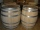Two cylinders are there one with oil and one with an empty oil cylinder has no fixed value assume infinitely. We are pumping out the oil into an empty cylinder having radius =1 cm height=3 cm rate of pumping oil is 9 cubic centimeters per sec and we are p
• Candles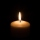Before Christmas, Eva bought two cylindrical candles - red and green. Red was 1 cm longer than green. She lit a red candle on Christmas Day at 5:30 p. M. , lit a green candle at 7:00 p. M. , and left them both on fire until they burned. At 9:30 p. M. , bo
• Cardboard box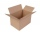Peter had square cardboard. The length of the pages was an integer in decimetres. He cut four squares with a side of 3 dm from the corners and made a box out of it, which fit exactly 108 cubes with an edge 1 dm long. Julia cut four squares with a side of
• Truncated coneCalculate the height of the rotating truncated cone with volume V = 794 cm3 and a base radii r1 = 9.9 cm and r2 = 9.8 cm.
• Pentagonal pyramidFind the volume and surface of a regular pentagonal pyramid with a base edge a = 12.8 cm and a height v = 32.1 cm.
• Angled cyclist turnThe cyclist passes through a curve with a radius of 20 m at 25 km/h. How much angle does it have to bend from the vertical inward to the turn?
• Mouse Hryzka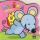Mouse Hryzka found 27 identical cubes of cheese. She first put in a large cube out of them and then waited for a while before the cheese cubes stuck together. Then from every wall of the big cube she will eats the middle cube. Then she also eats the cube
• PitThe pit has the shape of a truncated pyramid with a rectangular base and is 0.8 m deep. The pit's length and width are the top 3 × 1.5 m bottom 1 m × 0.5 m. To paint one square meter of the pit we use 0.6 l of green color. How many liters of paint are nee
• Equilateral coneWe pour so much water into a container that has the shape of an equilateral cone, the base of which has a radius r = 6 cm, that one-third of the volume of the cone is filled. How high will the water reach if we turn the cone upside down?
• Wooden blockA cuboid-shaped wooden block has 6 cm length, 4 cm breadth, and 1 cm height. Two faces measuring 4 cm x 1 cm are colored in black. Two faces measuring 6 cm x 1 cm are colored in red. Two faces measuring 6 cm x 4 cm are colored in green. The block is divid
• Top of the towerThe top of the tower has the shape of a regular hexagonal pyramid. The base edge has a length of 1.2 m, the pyramid height is 1.6 m. How many square meters of sheet metal is needed to cover the top of the tower if 15% extra sheet metal is needed for joint
• Brick wall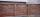Garden 70 m long and 48 m wide should surround with wall 2.1 meters high and 30 cm thick. Wall will be built on the garden ground. How many will we need bricks if to 1 m³ is required approximately 300 bricks?
• Eq2 2Solve following equation with quadratic members and rational function: (x2+1)/(x-4) + (x2-1)/(x+3) = 23
• Total displacementCalculate total displacement of the 4-cylinder engine with the diameter of the piston bore B = 6.6 cm and stroke S=2.4 cm of the piston. Help: the crankshaft makes one revolution while the piston moves from the top of the cylinder to the bottom and back t
• Rhombus constructionConstruct ABCD rhombus if its diagonal AC=9 cm and side AB = 6 cm. Inscribe a circle in it touching all sides...
• Four prismsQuestion No. 1: The prism has the dimensions a = 2.5 cm, b = 100 mm, c = 12 cm. What is its volume? a) 3000 cm2 b) 300 cm2 c) 3000 cm3 d) 300 cm3 Question No.2: The prism base is a rhombus with a side length of 30 cm and a height of 27 cm. The height of t

Do you have an interesting mathematical word problem that you can't solve it? Submit a math problem, and we can try to solve it.

We will send a solution to your e-mail address. Solved examples are also published here. Please enter the e-mail correctly and check whether you don't have a full mailbox.

Please do not submit problems from current active competitions such as Mathematical Olympiad, correspondence seminars etc...